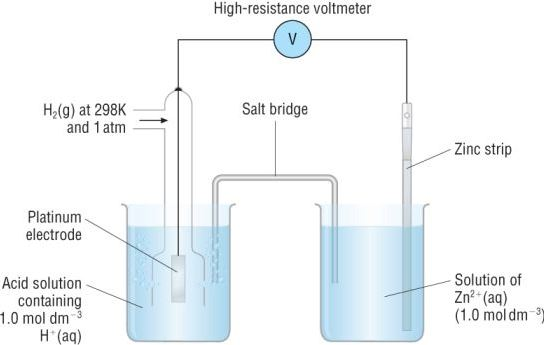# Standard Electrode Potential

Standard electrode potential is a measurement of the potential for equilibrium. There is a potential difference between the electrode and the electrolyte called the potential of the electrode. When unity is the concentrations of all the species involved in a semi-cell, the electrode potential is known as the standard electrode potential.

## Standard Electrode Potential Definition

Under standard conditions, the standard electrode potential occurs in an electrochemical cell say the temperature = 298K, pressure = 1atm, concentration = 1M. The symbol ‘Eocell’ represents the standard electrode potential of a cell.

## Significance of Standard Electrode Potential

• All electrochemical cells are based on redox reactions, which are made up of two half-reactions.
• The oxidation half-reaction occurs at the anode and it involves a loss of electrons.
• Reduction reaction takes place at the cathode, involving a gain of electrons. Thus, the electrons flow from the anode to the cathode.
• The electric potential that arises between the anode and the cathode is due to the difference in the individual potentials of each electrode (which are dipped in their respective electrolytes).
• The cell potential of an electrochemical cell can be measured with the help of a voltmeter. However, the individual potential of a half-cell cannot be accurately measured alone.
• It is also important to note that this potential can vary with a change in pressure, temperature, or concentration.
• In order to obtain the individual reduction potential of a half-cell, the need for standard electrode potential arises.
• It is measured with the help of a reference electrode known as the standard hydrogen electrode (abbreviated to SHE). The electrode potential of SHE is 0 Volts.
• The standard electrode potential of an electrode can be measured by pairing it with the SHE and measuring the cell potential of the resulting galvanic cell.
• The oxidation potential of an electrode is the negative of its reduction potential. Therefore, the standard electrode potential of an electrode is described by its standard reduction potential.
• Good oxidizing agents have high standard reduction potentials whereas good reducing agents have low standard reduction potentials.
• For example, the standard electrode potential of Ca+ is -3.8V and that of F2 is +2.87V. This implies that F2 is a good oxidizing agent whereas Ca is a reducing agent.

## Standard Electrode Potential Example

The calculation of the standard electrode potential of a zinc electrode with the help of the standard hydrogen electrode is illustrated below.It can be noted that this potential is measured under standard conditions where the temperature is 298K, the pressure is 1 atm, and the concentration of the electrolytes is 1M.

## Spontaneity of Redox Reactions

If a redox reaction is spontaneous, the ΔGo (Gibbs free energy) must have a negative value. It is described by the following equation:

ΔGocell = -nFE0cell

Where n refers to the total number of moles of electrons for every mole of product formed, F is Faraday’s constant (approximately 96485 C.mol-1).

The E0cell can be obtained with the help of the following equation:

E0cell = E0cathode – E0anode

Therefore, the E0cell can be obtained by subtracting the standard electrode potential of the anode from that of the cathode. For a redox reaction to be spontaneous, the E0cell must have a positive value (because both n and F have positive positive values, and the ΔGo value must be negative).

This implies that in a spontaneous process,

E0cell > 0; which in turn implies that E0cathode > E0anode

Thus, the standard electrode potential of the cathode and the anode help in predicting the spontaneity of the cell reaction. It can be noted that the ΔGo of the cell is negative in galvanic cells and positive in electrolytic cells.

## FAQs

1. What is electrode potential?
Ans: The potential of an electrode is known as the potential of a cell consisting of the electrode concerned acting as a cathode and the standard hydrogen electrode acting as an anode. The cathode is always reduced, and the anode is oxidized.

2. What is the difference between the standard electrode potential and standard cell potential?
Ans: In a class known as normal cell potential or standard electrode potential, the standard reduction potential is present. The natural potential of cells is the potential difference between the cathode and anode. All standard potentials are measured at 298 K, 1 atm and 1 M solutions.

3. What does electrode potential depend on?
Ans: An electrode’s tendency to lose electrons is called the potential for oxidation, while an electrode’s tendency to absorb electrons is called the potential for reduction. The potential for electrodes depends on metal ion concentration and temperature.

4. What factors affect cell potential?
Ans: Temperature, surface area, and concentration are the main factors influencing chemical reactions.

5. What increases cell potential?
Ans: Through increasing the concentration of one of the electrolyte solutions, you increase the number of cations and anions (depending on which electrolyte you increase), thus increasing the cell’s voltage potential.